Ex 14.2

Chapter 14 Class 7 Symmetry
Serial order wise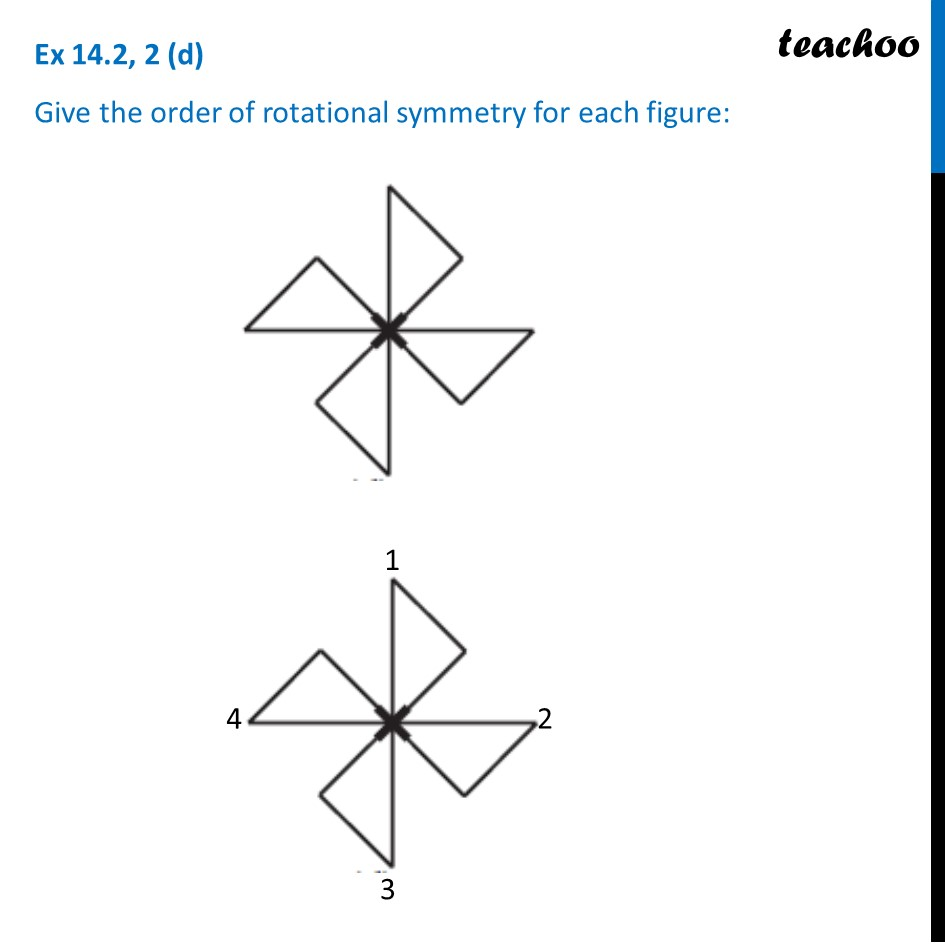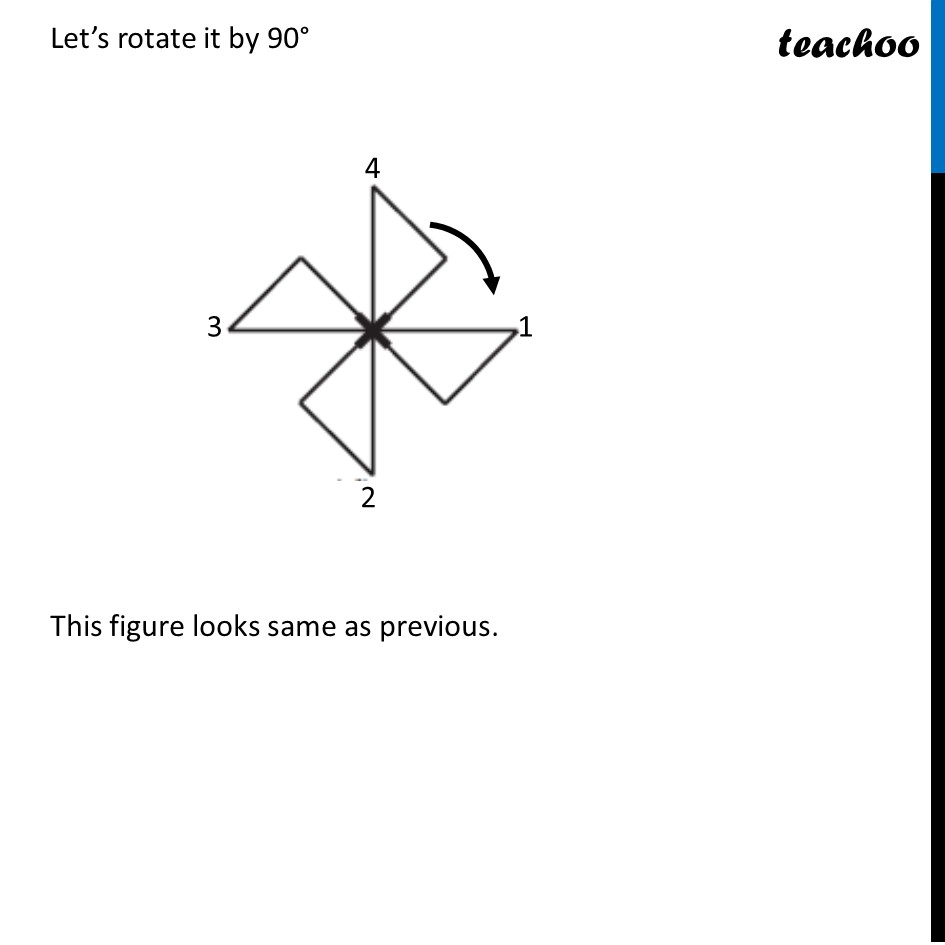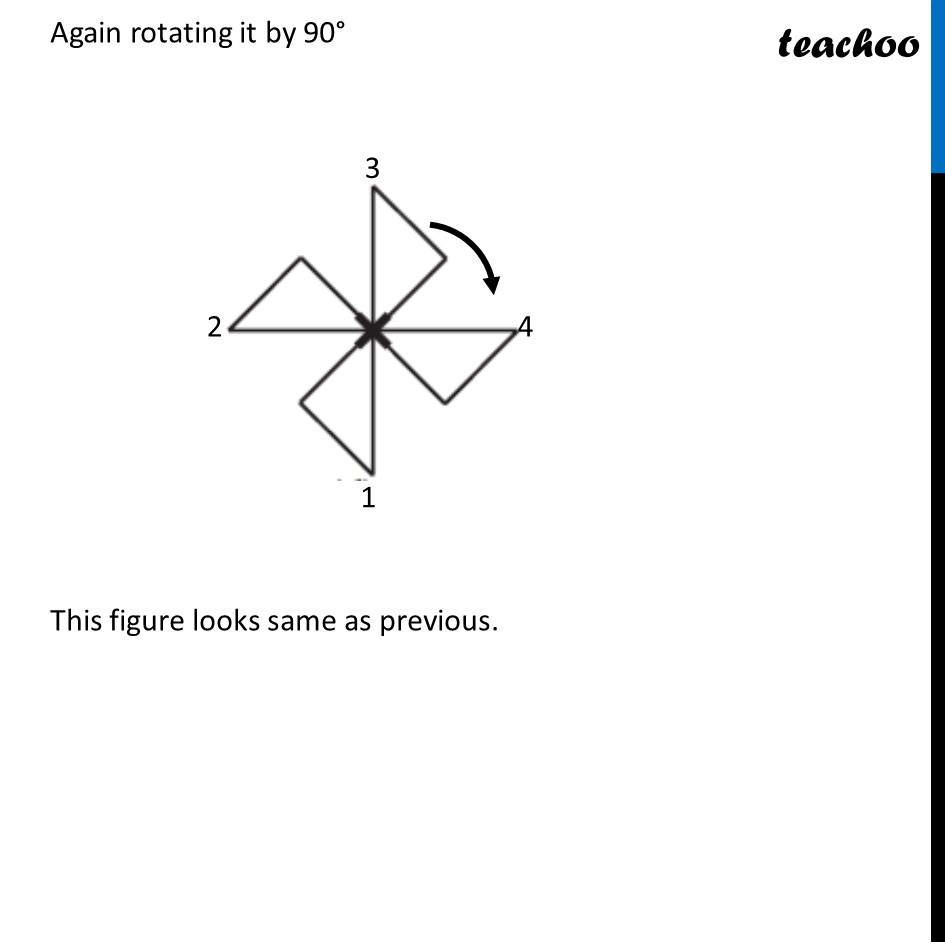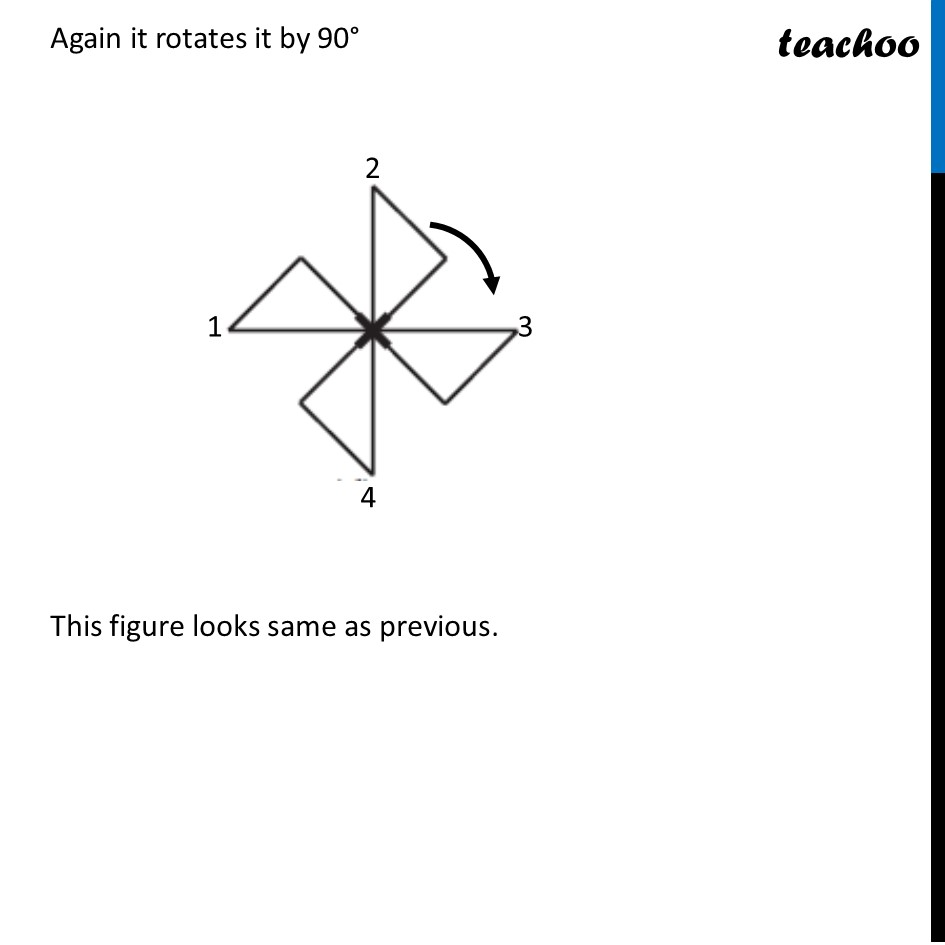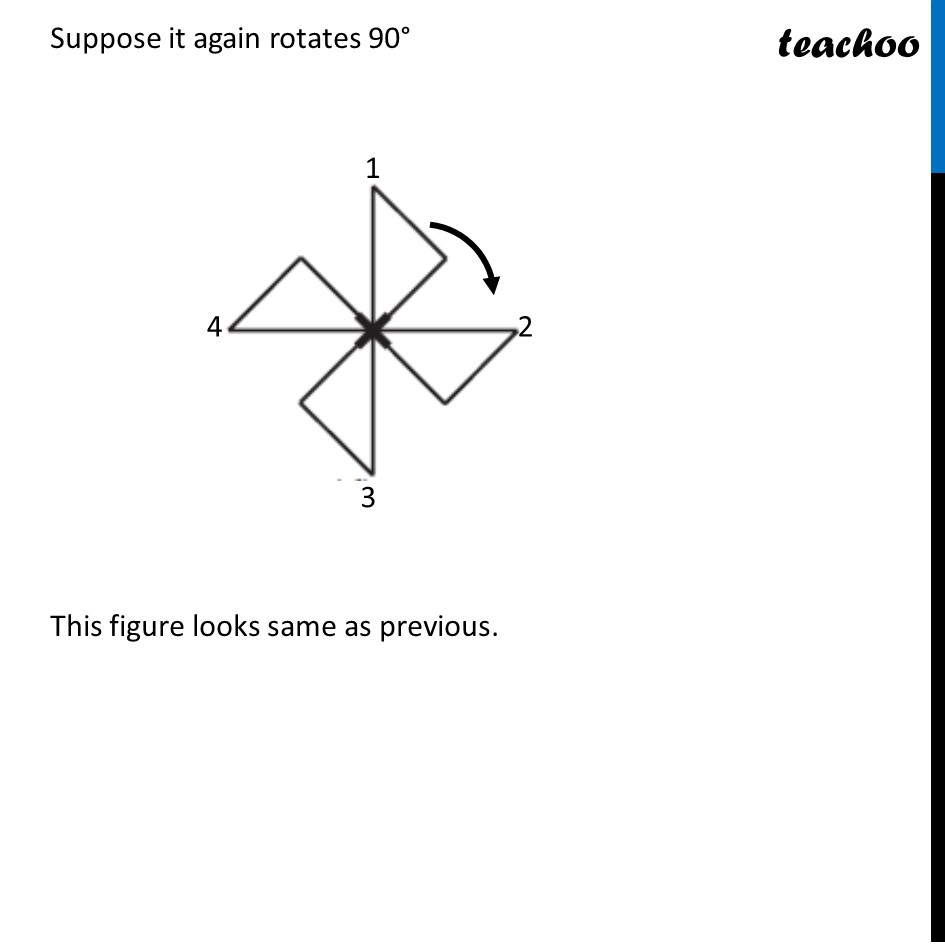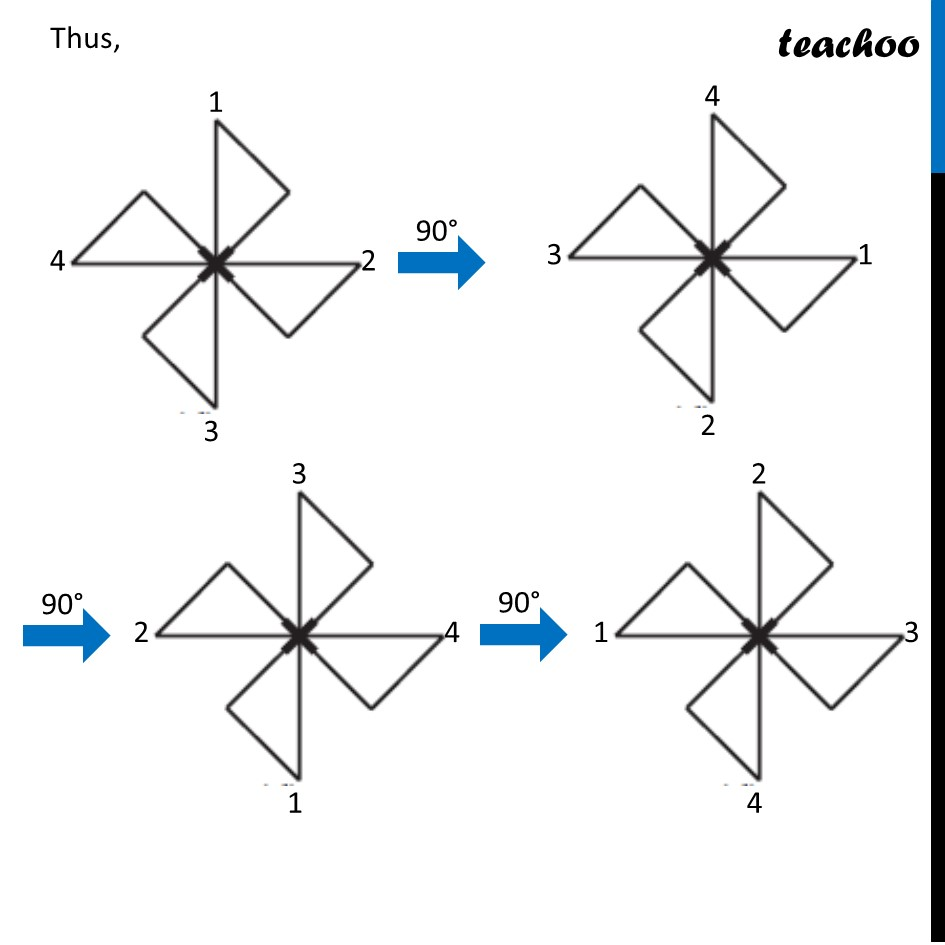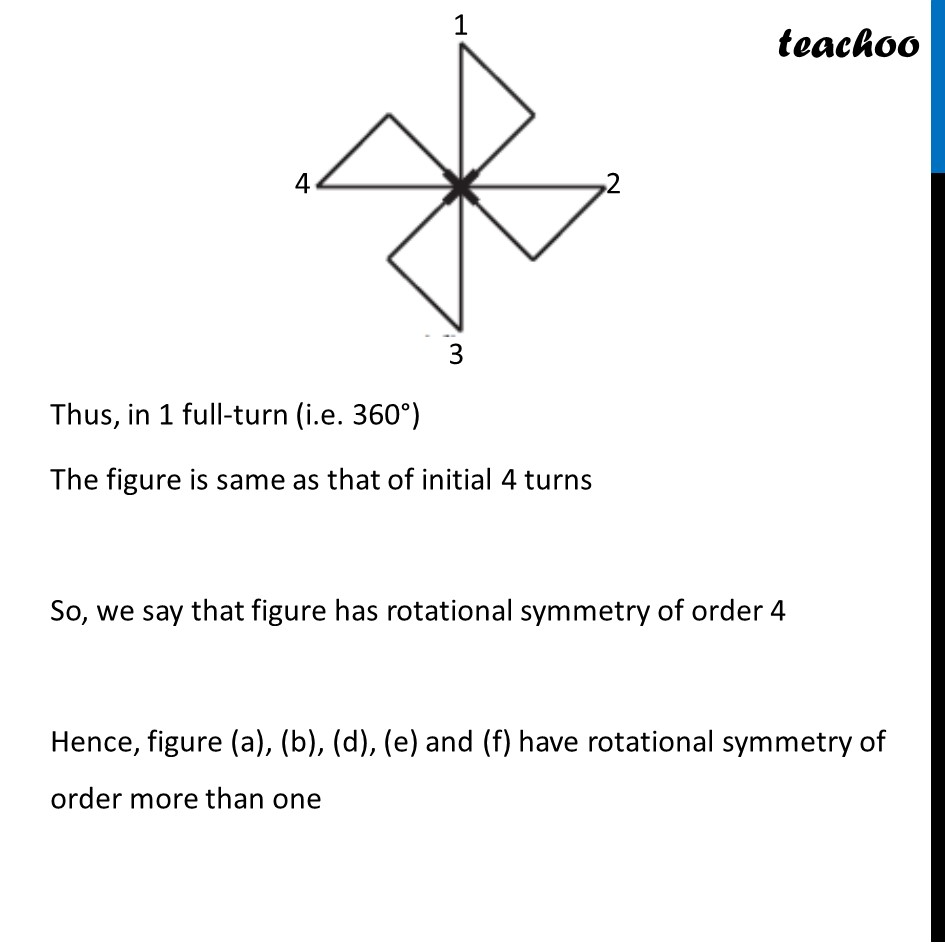Get live Maths 1-on-1 Classs - Class 6 to 12

### Transcript

Ex 14.2, 2 (d) Give the order of rotational symmetry for each figure: Let’s rotate it by 90° This figure looks same as previous. Again rotating it by 90° This figure looks same as previous. Again it rotates it by 90° This figure looks same as previous. Suppose it again rotates 90° This figure looks same as previous. Suppose it again rotates 90° This figure looks same as previous. Thus, Thus, in 1 full-turn (i.e. 360°) The figure is same as that of initial 4 turns So, we say that figure has rotational symmetry of order 4 Hence, figure (a), (b), (d), (e) and (f) have rotational symmetry of order more than one